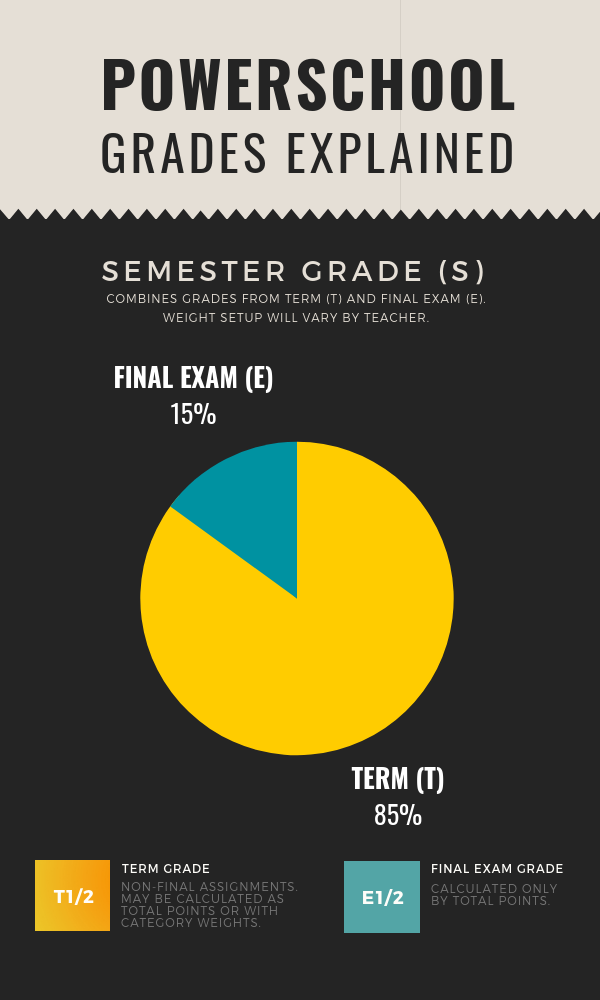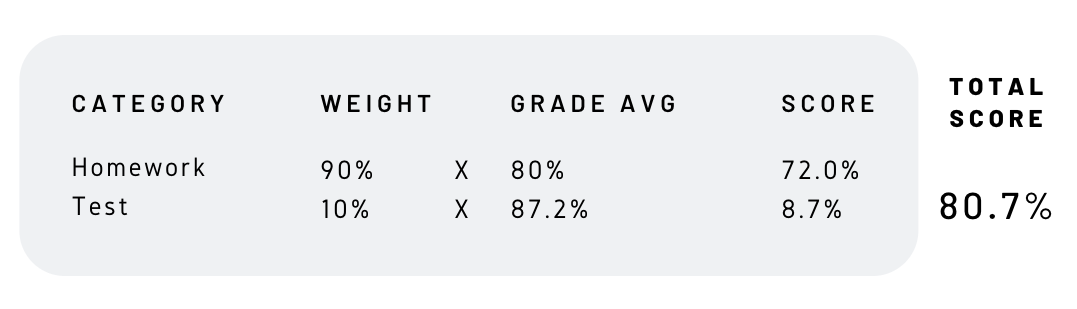Follow

Each O'Dowd teacher has their own way of calculating overall grades. Some teachers weight categories, others use total points, and many teachers have a final that is worth a certain percentage of the overall grade.

The diagram below demonstrates the breakdown of overall semester grades (S) - the numeric percentage values of semester (S), term (T), and final exam (E), which will vary from teacher to teacher.Q: What does it mean when my teachers says the "final will affect 15% of the overall semester grade"?

A: The semester grade is made up of the term grade (85%) and a final exam grade (15%). This means that if a student does very poorly on the final, the most percentage points the grade could drop would be 15.

Here is an example of grade breakdowns for a class that has a 15% final exam grade as well as categories within the term (non-final exam period).Q: My teacher calculates Term grades with a straight scale (i.e. total points). What does this mean?

A: Every point that is given for any assignment is equally weighted.

Example: A class has 6 assignments in the gradebook, 5 of which are 5-point Homework assignments, and 1 is a 55 point Test (80 points total). A student has earned 4/5 on all homework assignments, and a 48/55 on the test. The student has a B = 85.7% = (4+4+4+48)/70 = 60/70

Q: My teacher weights Term grades (i.e. calculates grades with category weights). What does this mean?

A: Every point that is given for every assignment are not equally weighted. I.e. Categories with higher weights will have greater emphasis on the grade those with lower weights.

Example: A class has category weights in which Tests are worth 10% and Homework is work 90% of the term grade. A student has received an average of 80% on all homework assignments, and a average of 87.2% on Tests. View the breakdown below to see how the student has earned a B- = 80.7%.A: This can happen when grades are weighted and if the score on the assignment is lower than the average for that category.

Example: Student’s overall grade is 90% for all categories (Test, Homework, Project) and they get a 92% on the next test. However if their test average is 100% then the weighted average for the Tests category will go down and therefore their overall grade will go down as well.

 BEFORE: Test (95/100)*25% + Homework (90/100)*25% + Project (88/100)*50% = = 90.25 AFTER entering 92% Test grade: Test (187/200)*25% + Homework (90/100)*25% + Project (88/100)*50% = = 89.88

Q: I don't get how such a small assignment could drop me a whole letter grade.

A: You may have a borderline grade.

Example: The student's current overall grade is 90.346%. In the Participation category, he starts out with 10/10=100%, but after receiving a 8.25/10=82.5%, his average participation score is now 18.25/20=92.5%. His weighted Participation category average will drop, thus lowering his overall grade.

 BEFORE: Participation (10/10)*10% + Homework (99.23)*20% + Tests (95)*30% + Projects (80)*40%= =10+19.846+28.5+32 =90.346 =A- AFTER entering 92.5% Participation grade: Participation (18.25/20)*10% + Homework (99.23)*20% + Tests (95)*30% + Projects (80)*40%= =9.13+19.846+28.5+32 =89.476 =B+